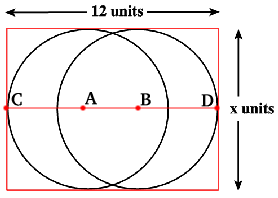#### You may also like### Square Pegs

Which is a better fit, a square peg in a round hole or a round peg in a square hole?### Cube Paths

Given a 2 by 2 by 2 skeletal cube with one route `down' the cube. How many routes are there from A to B?### Coins on a Plate

Points A, B and C are the centres of three circles, each one of which touches the other two. Prove that the perimeter of the triangle ABC is equal to the diameter of the largest circle.

# Not So Little X

##### Age 11 to 14Challenge Level

Two circles are enclosed by a rectangle 12 units by x units.

The distance between the centres of the two circles is x/3 units.

How big is x?